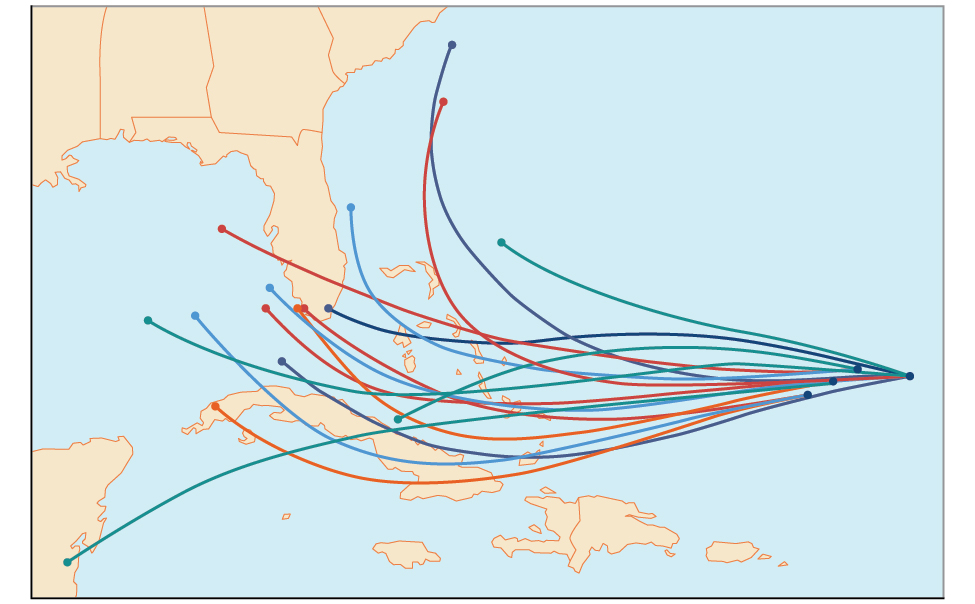# 13.7 Probability

 Page 1 / 18
In this section, you will:
• Construct probability models.
• Compute probabilities of equally likely outcomes.
• Compute probabilities of the union of two events.
• Use the complement rule to find probabilities.
• Compute probability using counting theory.An example of a “spaghetti model,” which can be used to predict possible paths of a tropical storm. The figure is for illustrative purposes only and does not model any particular storm.

Residents of the Southeastern United States are all too familiar with charts, known as spaghetti models, such as the one in [link] . They combine a collection of weather data to predict the most likely path of a hurricane. Each colored line represents one possible path. The group of squiggly lines can begin to resemble strands of spaghetti, hence the name. In this section, we will investigate methods for making these types of predictions.

## Constructing probability models

Suppose we roll a six-sided number cube. Rolling a number cube is an example of an experiment    , or an activity with an observable result. The numbers on the cube are possible results, or outcomes    , of this experiment. The set of all possible outcomes of an experiment is called the sample space    of the experiment. The sample space for this experiment is $\left\{1,2,3,4,5,6\right\}.\text{\hspace{0.17em}}$ An event    is any subset of a sample space.

The likelihood of an event is known as probability    . The probability of an event $p$ is a number that always satisfies $0\le p\le 1,$ where 0 indicates an impossible event and 1 indicates a certain event. A probability model    is a mathematical description of an experiment listing all possible outcomes and their associated probabilities. For instance, if there is a 1% chance of winning a raffle and a 99% chance of losing the raffle, a probability model would look much like [link] .

Outcome Probability
Winning the raffle 1%
Losing the raffle 99%

The sum of the probabilities listed in a probability model must equal 1, or 100%.

Given a probability event where each event is equally likely, construct a probability model.

1. Identify every outcome.
2. Determine the total number of possible outcomes.
3. Compare each outcome to the total number of possible outcomes.

## Constructing a probability model

Construct a probability model for rolling a single, fair die, with the event being the number shown on the die.

Begin by making a list of all possible outcomes for the experiment. The possible outcomes are the numbers that can be rolled: 1, 2, 3, 4, 5, and 6. There are six possible outcomes that make up the sample space.

Assign probabilities to each outcome in the sample space by determining a ratio of the outcome to the number of possible outcomes. There is one of each of the six numbers on the cube, and there is no reason to think that any particular face is more likely to show up than any other one, so the probability of rolling any number is $\text{\hspace{0.17em}}\frac{1}{6}.$

 Outcome Roll of 1 Roll of 2 Roll of 3 Roll of 4 Roll of 5 Roll of 6 Probability $\frac{1}{6}$ $\frac{1}{6}$ $\frac{1}{6}$ $\frac{1}{6}$ $\frac{1}{6}$ $\frac{1}{6}$

Do probabilities always have to be expressed as fractions?

No. Probabilities can be expressed as fractions, decimals, or percents. Probability must always be a number between 0 and 1, inclusive of 0 and 1.

#### Questions & Answers

write down the polynomial function with root 1/3,2,-3 with solution
if A and B are subspaces of V prove that (A+B)/B=A/(A-B)
write down the value of each of the following in surd form a)cos(-65°) b)sin(-180°)c)tan(225°)d)tan(135°)
Prove that (sinA/1-cosA - 1-cosA/sinA) (cosA/1-sinA - 1-sinA/cosA) = 4
what is the answer to dividing negative index
In a triangle ABC prove that. (b+c)cosA+(c+a)cosB+(a+b)cisC=a+b+c.
give me the waec 2019 questions
the polar co-ordinate of the point (-1, -1)
prove the identites sin x ( 1+ tan x )+ cos x ( 1+ cot x )= sec x + cosec x
tanh`(x-iy) =A+iB, find A and B
B=Ai-itan(hx-hiy)
Rukmini
what is the addition of 101011 with 101010
If those numbers are binary, it's 1010101. If they are base 10, it's 202021.
Jack
extra power 4 minus 5 x cube + 7 x square minus 5 x + 1 equal to zero
the gradient function of a curve is 2x+4 and the curve passes through point (1,4) find the equation of the curve
1+cos²A/cos²A=2cosec²A-1
test for convergence the series 1+x/2+2!/9x3ByBy Nick SwainByByByBy Rhodes